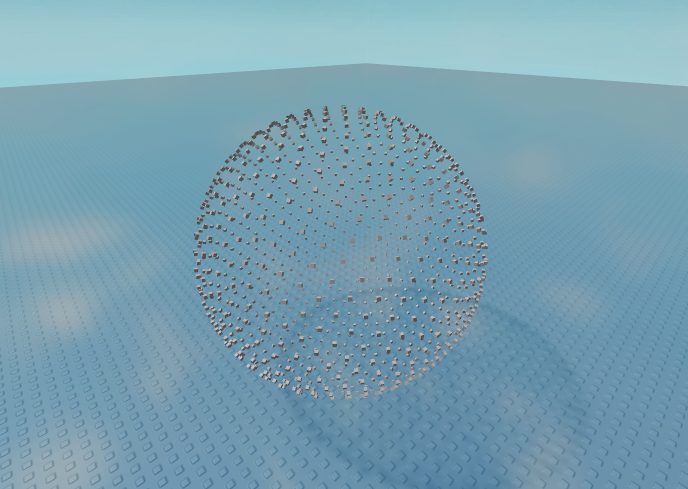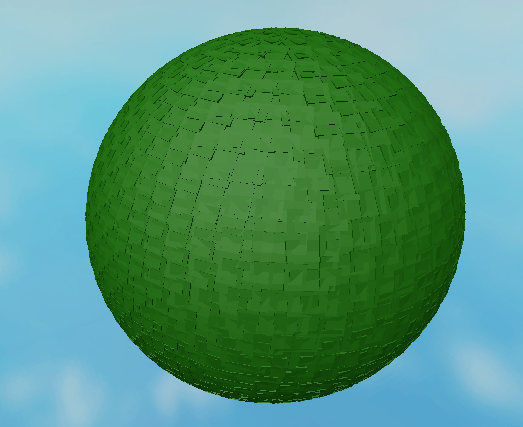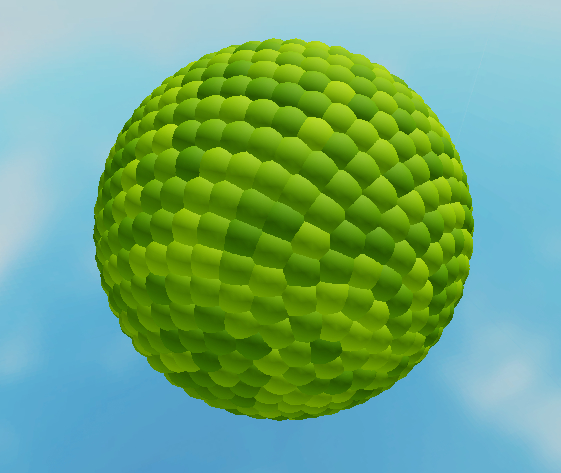# Using Math to generate Spheres

I still dont see how I can make a sphere out of this

That function returns an array of vectors that outline the surface of the sphere.

Here I placed parts on each position:

``````local function fibonacci_spiral_sphere(num_points)
local vectors = {}
local gr = (math.sqrt(5) + 1) / 2
local ga = (2 - gr) * (2 * math.pi)

for i = 1, num_points do
local lat = math.asin(-1 + 2 * i / (num_points + 1))
local lon = ga * i

local x = math.cos(lon) * math.cos(lat)
local y = math.sin(lon) * math.cos(lat)
local z = math.sin(lat)

table.insert(vectors, Vector3.new(x, y, z))
end

return vectors
end

local ref = Instance.new("Part")
ref.Anchored = true
ref.Size = Vector3.new(0.2, 0.2, 0.2)

local vec = fibonacci_spiral_sphere(1024)
for _, v in ipairs(vec) do
local part = ref:Clone()
part.Parent = workspace
wait()
end
``````

Result:Then it’s just a matter of resizing the nodes or connecting them to smooth out the surface.

8 Likes

every vector is a point in space: the function returns a list of points in space. Generate a “Ball” part with a size of 0.1, 0.1, 0.1 for each point, and set its position to that point.

So how would I calculate the Size and amount of points needed for a perfect enclosed sphere?

No idea. You would probably need some method of finding the closest points to any point and creating a triangle between them. That’s why my first solution was a geodesic sphere - I already knew where the triangles were and which points to connect.

This is so informative.
I was looking for this for a long time now but i forgot about it lol
Here is what I’ve done with it:2 Likes

How did you do that second circle?

1 Like

Do you know how I could turn this into terrain?

I increased the size (roughly 2 studs) and changed the shape of reference part to “Ball”
and to make a little variation in color. I just used math.random and Color3.fromRGB functions

Oh dip nice that is 2 studs longer btw

2 Likes

24 posts were split to a new topic: Using Math to generate Spheres (Private Discussion )